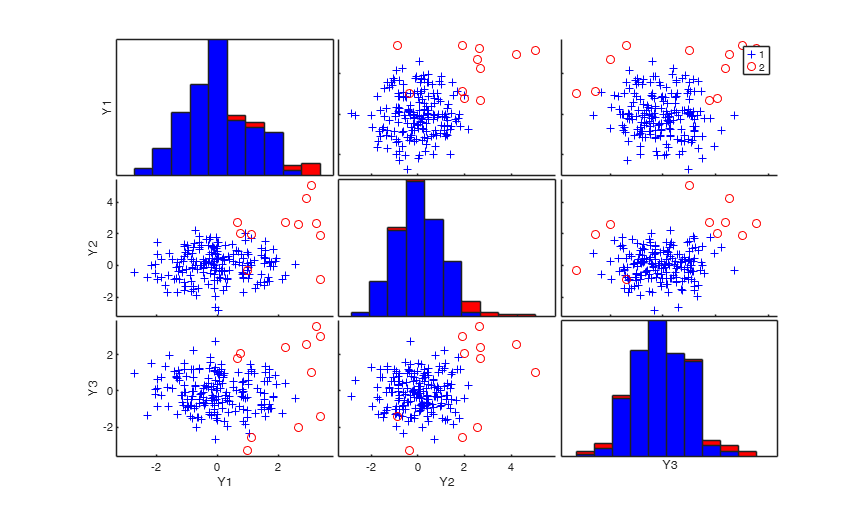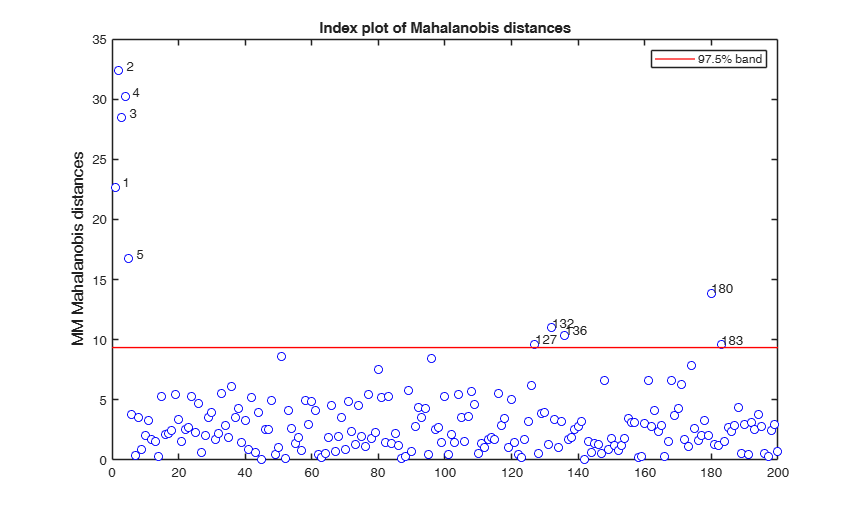MMmultcore

MMmultcore computes multivariate MM estimators for a selected fixed scale

Syntax

• out=MMmultcore(Y,loc0,shape0,auxscale)example
• out=MMmultcore(Y,loc0,shape0,auxscale,Name,Value)example

Description

out =MMmultcore(Y, loc0, shape0, auxscale) MMmultcore with all default options.

out =MMmultcore(Y, loc0, shape0, auxscale, Name, Value) MMmultcore with optional arguments.

Examples

expand all

MMmultcore with all default options.

Determine, e.g., an S estimate and extract the required arguments for the MM estimate.

n=200;
v=3;
randn('state', 123456);
Y=randn(n,v);
% Contaminated data
Ycont=Y;
Ycont(1:5,:)=Ycont(1:5,:)+3;
[out]=Smult(Ycont);
outMM=MMmultcore(Ycont,out.loc,out.shape,out.scale);MMmultcore with optional arguments.

Determine, e.g., an S estimate and extract the required arguments for the MM estimate.

n=200;
v=3;
randn('state', 123456);
Y=randn(n,v);
% Contaminated data
Ycont=Y;
Ycont(1:5,:)=Ycont(1:5,:)+3;
[out]=Smult(Ycont,'plots',1);
outMM=MMmultcore(Ycont,out.loc,out.shape,out.scale,'plots',1,'nocheck',1);
Total estimated time to complete S estimator:  1.06 secondsInput Arguments

Y — Input data. Matrix.

n x v data matrix; n observations and v variables. Rows of Y represent observations, and columns represent variables.

Missing values (NaN's) and infinite values (Inf's) are allowed, since observations (rows) with missing or infinite values will automatically be excluded from the computations.

Data Types: single|double

loc0 — initial estimate of location. Vector.

Vector containing initial estimate of location (generally an S estimate with high breakdown point, eg 0.5)

Data Types: single| double

shape0 — initial estimate of shape. Matrix.

v x v matrix containing initial estimate of shape (generally an S estimate with high breakdown point, eg 0.5)

Data Types: single| double

auxscale — initial estimate of scale. Scalar.

Scalar containing estimate of the scale (generally an S estimate with high breakdown point).

Data Types: single| double

Name-Value Pair Arguments

Specify optional comma-separated pairs of Name,Value arguments. Name is the argument name and Value is the corresponding value. Name must appear inside single quotes (' '). You can specify several name and value pair arguments in any order as Name1,Value1,...,NameN,ValueN.

Example: 'eff',0.99 , 'effshape',1 , 'refsteps',10 , 'tol',1e-10 , 'conflev',0.99 , 'plots',0 , 'nocheck',1 ,

eff —nominal efficiency.scalar.

Scalar defining nominal efficiency (i.e. a number between 0.5 and 0.99). The default value is 0.95 Asymptotic nominal efficiency is:

$(\int \psi' d\Phi)^2 / (\psi^2 d\Phi)$ For example if eff=0.95 and v=3, c=5.49 in case of location efficiency or c=6.096 in case of shape efficiency

Example: 'eff',0.99

Data Types: double

effshape —locacation or scale effiicency.dummy scalar.

If effshape=1 efficiency refers to shape efficiency else (default) efficiency refers to location

Example: 'effshape',1

Data Types: double

refsteps —Maximum iterations.scalar.

Scalar defining maximum number of iterations in the MM loop. Default value is 100.

Example: 'refsteps',10

Data Types: double

reftol —Tolerance.scalar.

Scalar controlling tolerance in the MM loop.

Default value is 1e-7

Example: 'tol',1e-10

Data Types: double

conflev —Confidence level which is used to declare units as outliers.scalar.

Usually conflev=0.95, 0.975 0.99 (individual alpha) or 1-0.05/n, 1-0.025/n, 1-0.01/n (simultaneous alpha).

Default value is 0.975

Example: 'conflev',0.99

Data Types: double

plots —Plot on the screen.scalar | structure.

If plots = 1, generates a plot of Mahalanobis distances against index number. The confidence level used to draw the confidence bands for the MD is given by the input option conflev. If conflev is not specified a nominal 0.975 confidence interval will be used.

Example: 'plots',0

Data Types: single | double

nocheck —Check input arguments.scalar.

If nocheck is equal to 1 no check is performed on matrix Y. As default nocheck=0.

Example: 'nocheck',1

Data Types: double

ysave —input data matrix Y is saved into the output structure out.scalar.

Scalar that is set to 1 to request that the data matrix Y is saved into the output structure out. This feature is meant at simplifying the use of function malindexplot.

Default is 0, i.e. no saving is done.

Example:

Data Types:

Output Arguments

out — description Structure

A structure containing the following fields

Value Description
loc

v x 1 vector. Estimate of location after refsteps refining steps

shape

v x v matrix. Estimate of the shape matrix after refsteps refining steps

cov

v x v matrix. Estimate of the covariance matrix after refsteps refining steps

md

n x 1 vector containing the estimates of the robust Mahalanobis distances (in squared units)

outliers

A vector containing the list of the units declared as outliers using confidence level specified in input scalar conflev

conflev

Confidence level that was used to declare outliers

weights

n x 1 vector. Weights assigned to each observation

class

'MM' out.Y : Data matrix Y. The field is present if option ysave is set to 1.

This routine does iterative reweighted least squares (IRWLS) steps from "initial location" (loc0) and shape matrix (shape0) keeping the estimate of the scale (auxscale) fixed.

Maronna, R.A., Martin D. and Yohai V.J. (2006), "Robust Statistics, Theory and Methods", Wiley, New York.

Acknowledgements

This function follows the lines of MATLAB/R code developed during the years by many authors.

For more details see http://www.econ.kuleuven.be/public/NDBAE06/programs/ and the R library robustbase http://robustbase.r-forge.r-project.org.

The core of these routines, e.g. the resampling approach, however, has been completely redesigned, with considerable increase of the computational performance.